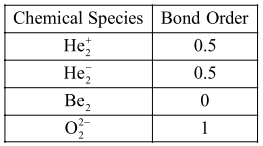# According to molecular theory,`
Question:

According to molecular theory, the species among the following that does not exist is:

1. $\mathrm{He}_{2}^{+}$

2. $\mathrm{He}_{2}^{-}$

3. $\mathrm{Be}_{2}$

4. $\mathrm{O}_{2}^{2-}$

Correct Option: , 3

Solution:According to M.O.T. If bond order of chemical species is zero then that chemical species does not exist.